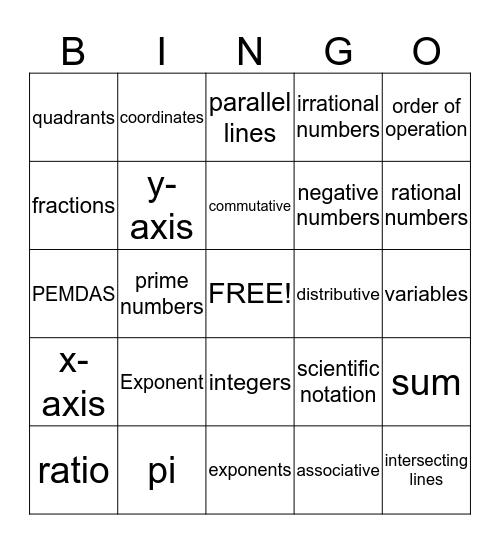# Untitled BingoThis bingo card has a free space and 24 words: Exponent, pi, commutative, ratio, order of operation, quadrants, fractions, coordinates, distributive, integers, negative numbers, PEMDAS, scientific notation, sum, y-axis, associative, variables, parallel lines, rational numbers, prime numbers, intersecting lines, x-axis, irrational numbers and exponents.

## Play Online